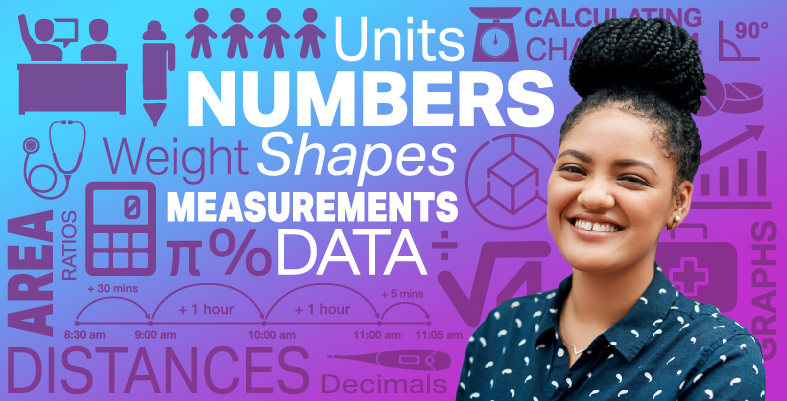Everyday maths for Health and Social Care and Education Support 2

Start this free course now. Just create an account and sign in. Enrol and complete the course for a free statement of participation or digital badge if available.

Free course

# 8.2 Formulas in practice

You will already have come across and used formulas in your everyday life. For example, if you are trying to work out how long to cook a fresh chicken for you may have used the formula:

Time (minutes) = 15 +  × 25 where ‘w’ is the weight of the chicken in grams.

For example, if you wanted to cook a chicken that weighs 2500 g you would do:

Time (minutes) = 15 + × 25

Remembering to use BIDMAS you would then get:

Time (minutes) = 15 + 5 × 25

= 15 + 125

= 140 minutes

Let’s look at another worked example before you try some on your own.

The owner of a guest house receives a gas bill. It has been calculated using the formula:

Cost of gas (£) =

Note: 8d means you do 8 × d.

Where d = number of days and u = number of units used

If she used 3500 units of gas in 90 days, how much is the bill?

In this example, d = 90 and u = 3500 so you do:

Cost of gas (£) =

=

=

= £42.20

## Activity 19: Using formulas

1. Fuel consumption in Europe is calculated in litres per 100 kilometres. A formula to approximate converting from miles per gallon to litres per 100 kilometres is:

• L =

Where L = number of litres per 100 kilometres and M = number of miles per gallon.

A car travels 40 miles per gallon. What is this in litres per kilometres?

2. You can convert temperatures from degrees Fahrenheit to degrees Celsius by using the formula:

• C =

Where C = temperature in degrees Celsius and F = temperature in degrees Fahrenheit.

If the temperature is 104 degrees Fahrenheit, what is the temperature in degrees Celsius?

1.

• L = and in this case M = 40

• L =

• L = 7 litres per 100 kilometres

2.

• C = and in this case F = 104

• C =

• C =

• C  =

• C = 40 degrees Celsius

Now that you have learned all the skills that relate to the number section of this course, there is just one final thing you need to be able to do before you will be ready to complete the end-of-session quiz for numbers.

You are now proficient at carrying out lots of different calculations including working out fractions and percentages of numbers, using ratio in different contexts and using formulas.

It is fantastic that you can now do all these things, but how do you check if an answer is correct? One way you can check would be to approximate an answer to the calculation (as you did in Section 2.2). Another way to check an answer is to use the inverse (opposite) operation.

## Summary

In this section you have:

• learned about, and practised using BIDMAS – the order in which operations must be carried out

• seen examples of formulas used in everyday life and practised using formulas to solve a problem.

FSM_SSH_2